#### Generic preprocessing phase

Figure 5.25 presents an outline of the basic preprocessing phase, and Figure 5.26 illustrates the algorithm. As seen throughout this chapter, the algorithm utilizes a uniform, dense sequence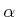. In each iteration, the algorithm must check whether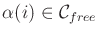. If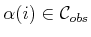, then it must continue to iterate until a collision-free sample is obtained. Once, then in line 4 it is inserted as a vertex of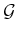. The next step is to try to connect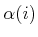to some nearby vertices,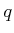, of. Each connection is attempted by the CONNECT function, which is a typical LPM (local planning method) from Section 5.4.1. In most implementations, this simply tests the shortest path betweenand. Experimentally, it seems most efficient to use the multi-resolution, van der Corput-based method described at the end of Section 5.3.4 . Instead of the shortest path, it is possible to use more sophisticated connection methods, such as the bidirectional algorithm in Figure 5.24. If the path is collision-free, then CONNECT returns TRUE.

The same_component condition in line 6 checks to make sureandare in different components ofbefore wasting time on collision checking. This ensures that every time a connection is made, the number of connected components ofis decreased. This can be implemented very efficiently (near constant time) using the previously mentioned union-find algorithm [243,823]. In some implementations this step may be ignored, especially if it is important to generate multiple, alternative solutions. For example, it may be desirable to generate solution paths from different homotopy classes. In this case the condition (not.same_component(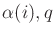)) is replaced with.vertex_degree()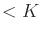, for some fixed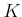(e.g., K = 15).

Steven M LaValle 2020-08-14